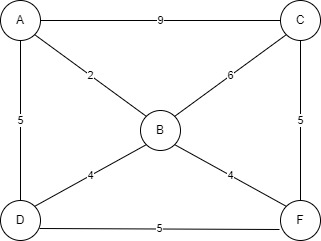Tribhuvan University

Institute of Science and Technology

2079

Bachelor Level / third-semester / Science

Computer Science and Information Technology( CSC206 )

Data Structure and Algorithm

Full Marks: 60 + 20 + 20

Pass Marks: 24 + 8 + 8

Time: 3 Hours

Candidates are required to give their answers in their own words as far as practicable.

The figures in the margin indicate full marks.

Section A

Attempt any two questions.

1

Why do we need to balance the binary search tree? Justify with an example. Create an AVL tree from the data 24, 12, 8, 15, 35, 30, 57, 40, 45, 78.

2

How recursive algorithm uses stack to store intermediate results? Illustrate with an example. Convert the infix expression  A+B-(C*D/E+F)-G*H into postfix expression using stack.

3

How do you insert and delete a node at kth position of the doubly linked list? Describe the process of implementing stack and queue using linked list.

Section B

Attempt any eight questions.

4

Sort the numbers 82,73,12,39,26,88,2,9,60,41 using shell sort.

5

Why do we need asymptotic notation? Describe about Big oh notation with its curve.

6

Define queue. Explain about enqueue and dequeue operation in circular queue.

7

Write a program to implement binary search.

8

Find the MST of following graph using Prim’s algorithm.9

Assume you have to store the data {0,1,2,4,5,7} into a hash table of size 5, with hash function, h(x)=x%5. Apply linear probing and double hashing as collision resolution techniques.

10

In which case the position of pivot element in quick sort always either in the last or the first position? Create a max heap from the numbers. {10,12,53,34,23,77,59,66,5,8}

11

Evaluate the postfix expression 574-*8/4+ using stack.

12

Write short noes on:

a. Priority Queue

b. Breadth First traversal of a graph